## September 4, 2023

### Concurrent Process Histories and Resource Transducers

#### Posted by Emily Riehlguest post by Clémence Chanavat and Luke Morris

Let’s motivate this blog post by first formalizing what a resource theory is. Coecke et al. represent a resource theory in “A mathematical theory of resources” as a symmetric monoidal category (SMC), which is a familiar construction in applied category theory. Elegantly, each of the concepts used in defining a symmetric monoidal category has a simple physical intuition:

• Objects are resources
• Morphisms transform resources to other resources
• Composition is sequential transformation
• Tensoring is parallel transformation
• The unit is the “void resource”

Using the usual string diagram approach, we get diagrams representing resource transformations for free! We thus have a very expressive visual language for expressing resource transformations, and a familiar ACT formalization.

The most immediate shortcoming arises when we want to talk about concurrent processes. Although we can talk about “vertical” transformations forward in time, we want to now talk about “horizontal” transformations between parties, concurrently. As Nester puts it, we want resource “transducers”.

Taking a software engineer’s approach, let’s approach this problem by looking at what components we need to fill in and how we need to link them together. We have a way to encode process histories as SMCs already. We want:

1. to find some way to formalize resource transducers, and
2. to find some way to gather these together into a single mathematical object.

Let’s fill in #2 first. An ideal solution should be a familiar ACT construction, and should exhibit both “horizontal” and “vertical” notions of composition. A nice candidate is the single-object double category. A single-object double category has:

• A horizontal edge monoid $D_H$
• A vertical edge monoid $D_V$
• Cells with top and bottom boundaries from $D_H$, and left and right boundaries from $D_V$.

Now for #1. An ideal solution should be a familiar ACT construction, and should capture some notion of passing things/ polarity. We can introduce polarity by taking our resource theory, and making a copy of it for Alice and a copy for Bob. That is, for a resource theory $A$, we take $A \times \{\circ, \bullet\}$. To denote a series of exchanges, we can take the free monoid of $A \times \{\circ, \bullet\}$. These exchanges should satisfy some simple constraints to accurately model resource transducers, which we will get into immediately:

## The horizontal free cornering

Let $\mathbb{A}$ be a resource theory. The horizontal free cornering $\mathbf{H} \mathbb{A}$ is a way to introduce concurrency in our model. Any exchange $X_1 \otimes \cdots \otimes X_n$ will now involve a left (say Alice) and a right (say Bob) participant. The category $\mathbf{H} \mathbb{A}$ has objects $A^\circ$ and $A^\bullet$, for $A \in \mathbb{A}$, with interpretation that an element $A^\circ$ is the resource $A$ being sent from Alice to Bob, while $A^\bullet$ is $A$ going from Bob to Alice. These exchanges happen in order, $A^\circ \otimes B^\bullet$ is first Alice giving $A$ to Bob, then Bob giving $B$ to Alice. A morphism $h : X \to Y$ in this category is a transducer, operated on the domain by Alice and on the codomain by Bob. For instance, consider the morphism:To operate the transducer, Alice must supply water and will receive bread, while Bob must supply flour, receive dough, and finally supply bread. In this category, an isomorphism is to be though as an equivalent exchange, i.e. a sequence of event with the same end result. With this intuition in mind, we have the following expected results:

1. $I^\circ \simeq I \simeq I^\bullet$, exchanging nothing is the same as doing nothing;
2. $A^\circ \otimes B^\circ \simeq B^\circ \otimes A^\circ$ and $A^\bullet \otimes B^\bullet \simeq B^\bullet \otimes A^\bullet$, it does not matter in which order Alice gives items to Bob, and vice versa;
3. $(A\otimes B)^\circ \simeq A^\circ \otimes B^\circ$ and $(A\otimes B)^\bullet \simeq A^\bullet \otimes B^\bullet$, giving away a collection is giving away its components.

Moreover, there is a parametrized adjunction between giving to the left, and giving to the right, that is, for all $A \in \mathbb{A}$, we have

$A^\circ \otimes - \dashv A^\bullet \otimes - .$ The proof is by yanking, once we defined $\eta_X$ and $\varepsilon_X$, respectively by: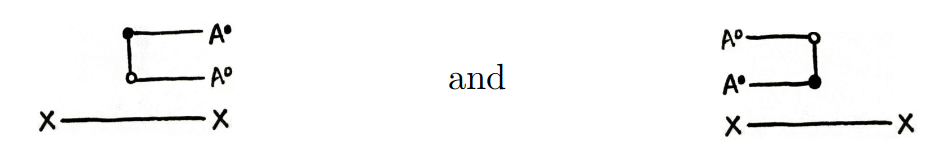we can prove the triangular identities by yanking two times, as in: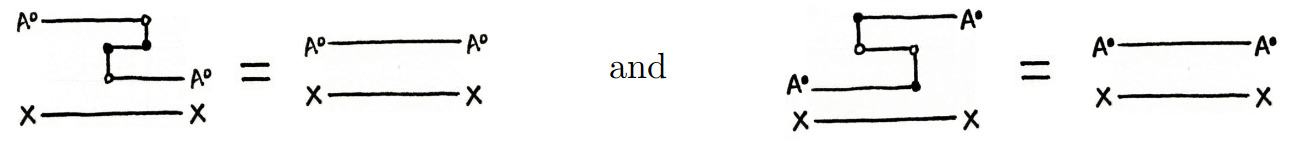On top of this duality, we have the fact that if we receive some resources before we are required to send any, then we can send some of the resources that we receive, however, if we must send the resource first, this is no longer possible. In short, we have a morphism $d^\circ_\bullet : A^\circ \otimes B^\bullet \to B^\bullet \otimes A^\circ,$ that need not to be an isomorphism. This reflects some sort of causal structure of $\mathbf{H} \mathbb{A}$: sending and receiving do not commute, but sending and sending always do, as we saw in the point 2. above. Note that this asymmetry stem from the fact that Alice operates the transducers on the left and Bob on the right.

To conclude, we give hints on how to present axiomatically $\mathbf{H} \mathbb{A}$. We create a category by first freely adding objects $A^\circ$ and $A^\bullet$ for each $A \in \mathbb{A}$, and morphisms $f^\circ : A^\circ \to B^\circ$, $f^\bullet : A^\bullet \to B^\bullet$, for each $f : A \to B$ in $\mathbb{A}$. Then, we add structural morphisms, that are split in two groups: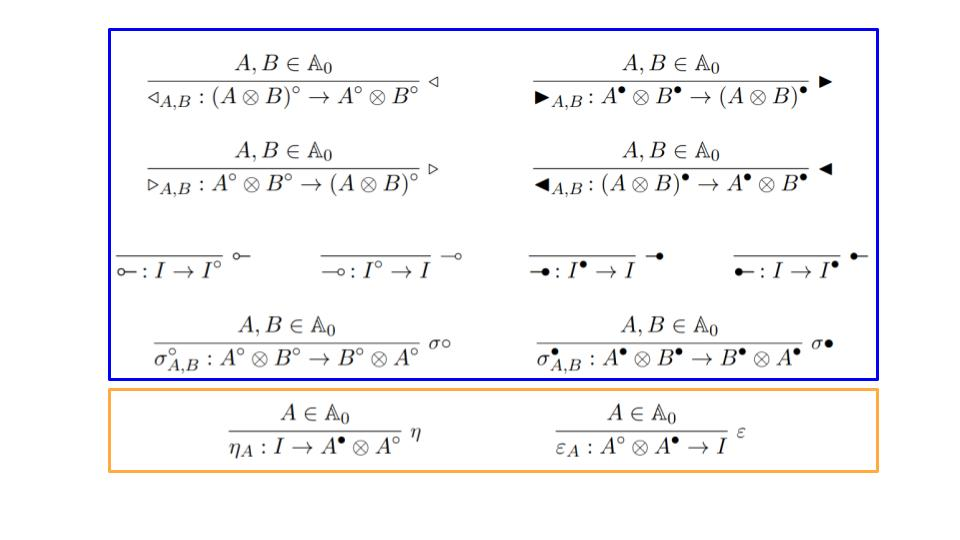The ones in the blue box correspond to the isomorphisms specified above, while the two in the orange box to the parametrized adjunctions $A^\circ \otimes - \dashv A^\bullet \otimes -$. Once we have this free structure, we quotient by (a lot) of equations. To see them more easily, we give a graphical calculus: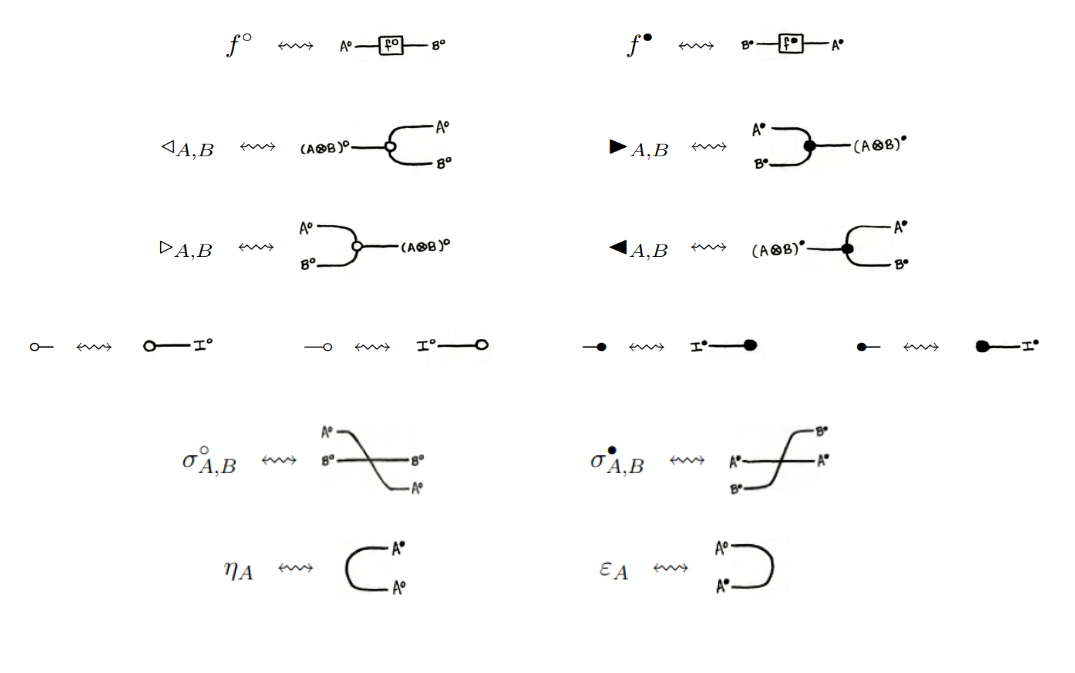and we specify interactions between all these morphisms. For instance, we require: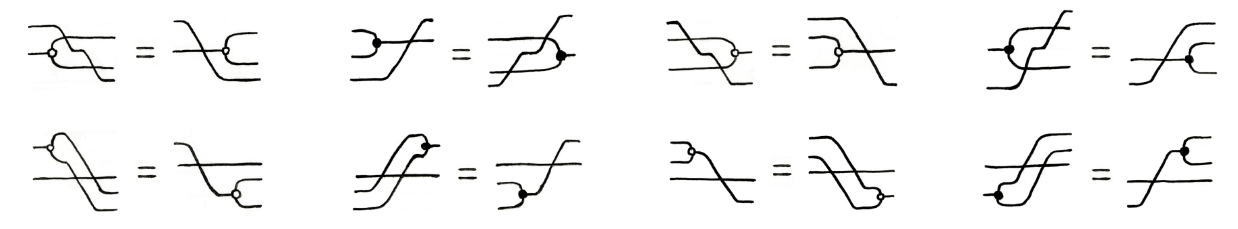Let us take a specific example: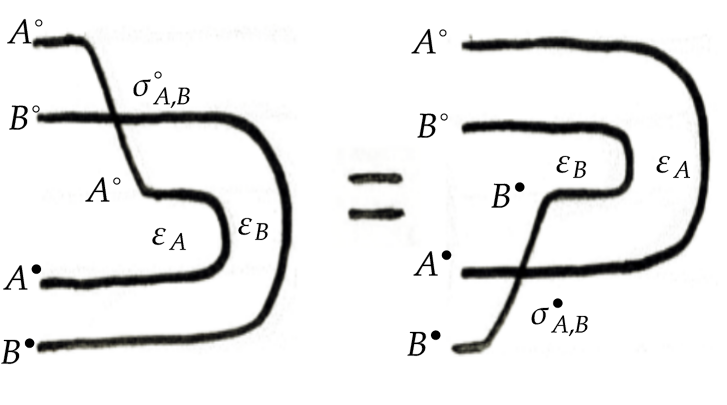It means that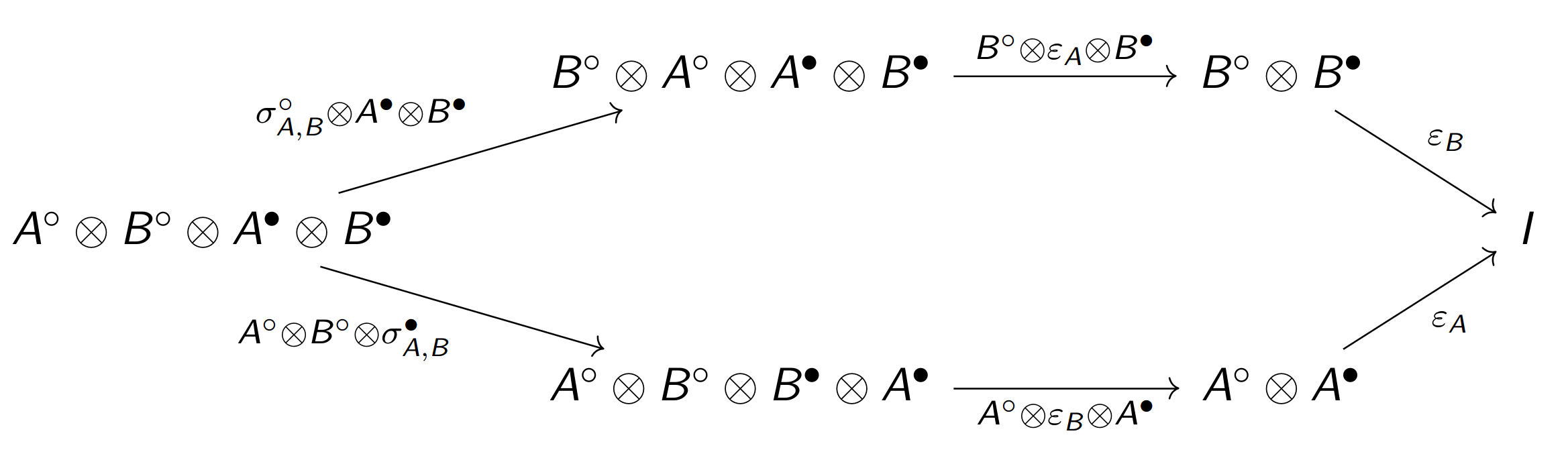commutes, i.e. that given an exchange $A^\circ \otimes B^\circ \otimes A^\bullet \otimes B^\bullet$, the two ways of getting to the identity by either

1. swapping $A^\circ \otimes B^\circ$, then annihilating $A^\circ \otimes A^\bullet$ and $B^\circ \otimes B^\bullet$ with the counit;
2. or, swapping $A^\bullet \otimes B^\bullet$, then annihilating $B^\circ \otimes B^\bullet$ and $A^\circ \otimes A^\bullet$ with the counit,

give the same end result, hence should give an equality of morphisms in the category. Thus, this free monoidal category quotiented by all the relations can be shown to be isomorphic to $\mathbf{H} \mathbb{A}$.

## Conclusion

We have seen how the Nester framework for resource transducers makes use of SMCs and single-object double categories. We then studied how the horizontal free cornering represents concurrency, and gave an axiomatic presentation of this construction. The next step taken by Nester in his latest paper “Protocol Choice and Iteration for the Free Cornering” is to extend the free cornering to support branching communication protocols and iterated communication protocols, by means of coproducts.

Posted at September 4, 2023 10:16 PM UTC

TrackBack URL for this Entry:   https://golem.ph.utexas.edu/cgi-bin/MT-3.0/dxy-tb.fcgi/3492